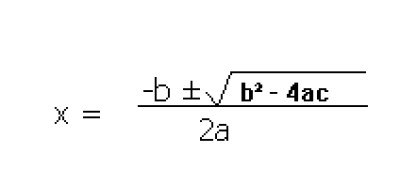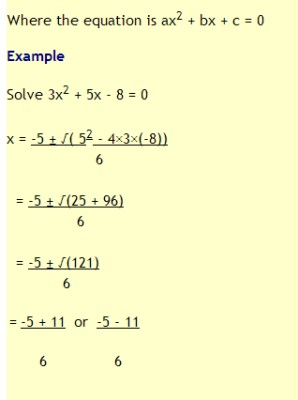List of public pages created with Protopage

# Home

## Plain sticky notes

### Hello

Hi, This is my webpage for maths revision and feel free to use.

## Calendars

### Calendar

• Tue November 27 - My Birthday ^_^

# Unit A

## Plain sticky notes

### Sin, Cos and Tan

In any right angled triangle, for any angle: The sine of the angle = the length of the opposite side divide by the length of the hypotenuse The cosine of the angle = the length of the adjacent side divide by the length of the hypotenuse The tangent of the angle = the length of the opposite side divide by the length of the adjacent side The hypotenuse of a right angled triangle is the longest side, which is the one opposite the right angle. The adjacent side is the side which is between the angle in question and the right angle. The opposite side is opposite the angle in question. sin = o/h cos = a/h tan = o/a Often remembered by: soh cah toa

### Pythagoras' Theorem

Pythagoras's Theorem In any right-angled triangle, the square of the hypotenuse is equal to the sum of the squares of the other two sides.

# Unit B

## Plain sticky notes

### Rounding to Decimal places

5 or more, we 'round up'. 4 or less, it stays as it is.

### Fraction/Decimals/Percentages

Fraction/Decimals/Percentages These are the 3 ways of expressing a part of a whole. How to converting them? http://www.youtube.com/watch?v=pCWKzbvTOKY

### Surds

A surd is a square root which cannot be reduced to a whole number. For example, is not a surd, as the answer is a whole number. But is not a whole number. You could use a calculator to find that but instead of this we often leave our answers in the square root form, as a surd. You need to be able to simplify expressions involving surds. Here are some general rules that you will need to learn. Here is a video clip about surds http://www.youtube.com/watch?v=D3SZE6zkQlM

# Unit C

## Plain sticky notes

### Standard Form

Standard form is a way of writing down very large or very small numbers easily. 103 = 1000, so 4 × 103 = 4000 . So 4000 can be written as 4 × 10³ . This idea can be used to write even larger numbers down easily in standard form. Small numbers can also be written in standard form. However, instead of the index being positive (in the above example, the index was 3), it will be negative. The rules when writing a number in standard form is that first you write down a number between 1 and 10, then you write × 10(to the power of a number). Example: Write 81 900 000 000 000 in standard form: 81 900 000 000 000 = 8.19 × 1013 It’s 1013 because the decimal point has been moved 13 places to the left to get the number to be 8.19

A quadratic equation is an equation where the highest power of x is x2. There are various methods of solving quadratic equations, as shown below. Important point about square roots: 62 = 36. But also (-6)2 = 36 because -6 × -6 = + 36 (a minus × a minus = a plus). Therefore there are two square roots of 36: +6 and -6. We call 6 the positive square root of 36 and -6 is called the negative square root of 36. So if x2 = 36, then x = +6 or -6 (since squaring either of these numbers will give 36). However, the square root sign means "positive square root". So √36 = + 6 (only).

### Probability

Probability is the likelihood or chance of an event occurring. Probability = the number of ways of achieving success/the total number of possible outcomes For example, the probability of flipping a coin and it being heads is ½, because there is 1 way of getting a head and the total number of possible outcomes is 2 (a head or tail). We write P(heads) = ½ . The probability of something which is certain to happen is 1. The probability of something which is impossible to happen is 0. The probability of something not happening is 1 minus the probability that it will happen.

## Photos